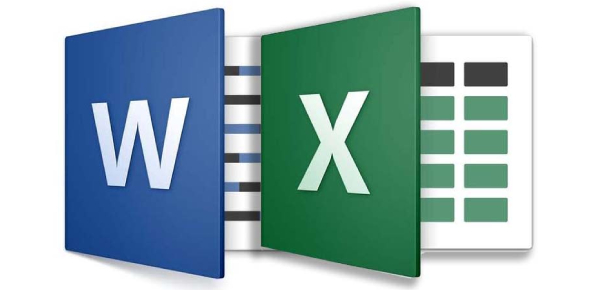# MS Word And Excel Test: Trivia Quiz!

25 Questions | Total Attempts: 6698SettingsCreate your own QuizMicrosoft word is one of the most used Microsoft applications, and you cannot claim to know anything about using a computer and not know a thing or two about Microsoft word. Are you looking for a way to test out your basic skills on this application? Take up the quiz below and get to see if you can tackle it. All the best of luck!

• 1.
The process of turning the system on is called?
• A.

Oning

• B.

Starting

• C.

Booting

• D.

Hard start

• 2.
What is not a basic part of a spreadsheet?
• A.

Formula

• B.

Cell

• C.

Row

• D.

Column

• 3.
Which of the following is not an input device?
• A.

Laptop

• B.

VDU

• C.

UPS

• D.

Mouse

• 4.
In a spreadsheet, the ________ is defined as the horizontal space that is going across the window.
• 5.
In a spreadsheet, the __________ is defined as the vertical space that is going across the window.
• 6.
_____________ are used to designate each column's location.
• 7.
___________ are used to designate each row's location.
• 8.
In a spreadsheet, the ___________ is defined as the space where a specified row and column intersect.
• A.

Labels

• B.

Cell

• C.

Formulas

• D.

Numbers

• 9.
Name this cell:
• 10.
In the spreadsheet below, provide an example of a label?
• 11.
True or False: Labels do not have a value associated with them
• A.

True

• B.

False

• 12.
True or False: Constants are entires that do not have a specified value
• A.

True

• B.

False

• 13.
In the spreadsheet below, provide an example of a constant:
• 14.
All formulas must begin with what?
• 15.
____________  are entires that have equations that calculates the value to display. n
• A.

Labels

• B.

Constants

• C.

• D.

Formulas

• 16.
Provide the formula for the following spreadsheet: If you work for 23 hours and make \$5.36 an hour, how much do you make?
• 17.
The ___________ function takes all of the values in each of the specified cells and totals their values
• 18.
The _________ function adds all of the indicated cells together and divides by the total number of cells.
• 19.
The two most widely used charts are the:
• 20.
Name the three basic parts of a spreadsheet?
• 21.
In a spreadsheet, each cell may have three types of data in them: Name all three
• 22.
What is the symbol for multiplication?
• 23.
What is the symbol for division?
• 24.
What is the symbol for addition?
• 25.
What is the symbol for subtraction?
Related TopicsBack to top
×

Wait!
Here's an interesting quiz for you.